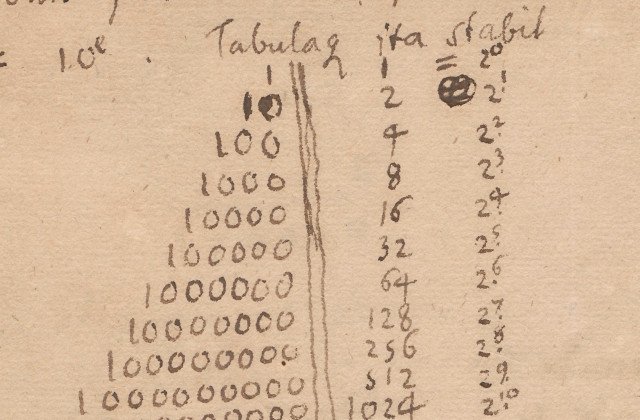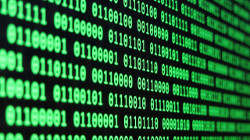# Bitwise operators [cheat sheet]

yourbasic.org/golangGottfried Wilhelm Leibniz, 1697

## Number literals

The binary number 100002 can be written as `020`, `16` or `0x10` in Go.

Literal Base Note
`020` 8 Starts with `0`
`16` 10 Never starts with `0`
`0x10` 16 Starts with `0x`

## Built-in operators

Operation Result Description
`0011 & 0101` 0001 Bitwise AND
`0011 | 0101` 0111 Bitwise OR
`0011 ^ 0101` 0110 Bitwise XOR
`^0101` 1010 Bitwise NOT (same as `1111 ^ 0101`)
`0011 &^ 0101` 0010 Bitclear (AND NOT)
`00110101<<2` 11010100 Left shift
`00110101<<100` 00000000 No upper limit on shift count
`00110101>>2` 00001101 Right shift
• The binary numbers in the examples are for explanation only. Integer literals in Go must be specified in octal, decimal or hexadecimal.
• The bitwise operators take both signed and unsigned integers as input. The right-hand side of a shift operator, however, must be an unsigned integer.
• Shift operators implement arithmetic shifts if the left operand is a signed integer and logical shifts if it is an unsigned integer.

## Package `math/bits`

Operation Result Description
`bits.UintSize` 32 or 64 Size of a uint in bits
`bits.OnesCount8(00101110)` 4 Number of one bits (population count)
`bits.Len8(00101110)` 6 Bits required to represent number
`bits.Len8(00000000)` 0
`bits.LeadingZeros8(00101110)` 2 Number of leading zero bits
`bits.LeadingZeros8(00000000)` 8
`bits.TrailingZeros8(00101110)` 1 Number of trailing zero bits
`bits.TrailingZeros8(00000000)` 8
`bits.RotateLeft8(00101110, 3)` 01110001 The value rotated left by 3 bits
`bits.RotateLeft8(00101110, -3)` 11000101 The value rotated right by 3 bits
`bits.Reverse8(00101110)` 01110100 Bits in reversed order
`bits.ReverseBytes16(0x00ff)` `0xff00` Bytes in reversed order
• The functions operate on unsigned integers.
• They come in different forms that take arguments of different sizes. For example, `Len`, `Len8`, `Len16`, `Len32`, and `Len64` apply to the types `uint`, `uint8`, `uint16`, `uint32`, and `uint64`, respectively.
• The functions are recognized by the compiler and on most architectures they are treated as intrinsics for additional performance.

## Bit manipulation code exampleBitmasks, bitsets and flags shows how to implement a bitmask, a small set of booleans, often called flags, represented by the bits in a single number.